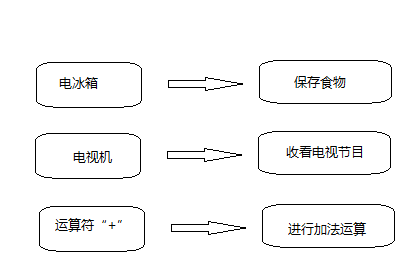C++学习笔记之三（C++语句基础之表达式与语句）解析

运算符概述：

C++语言中的运算符是可以让C++编译器能够识别的具有运算意义的符合。编译器把这些符合及组成的表达式翻译成相应的机器代码，就可以由计算机运行出正确的结果。如下图的表示：C++中包含了C语言中所有的运算符，并且在其基础上又增加了一下几种新的运算符。

：：:作用域运算符

new:动态分配内存运算符

delete:删除动态分配内存单元运算符

*和→:成员指针选择运算符。

重点注意：不同的运算符所需制定的操作数的个数不同，根据运算符需要的操作符的个数，可以分为三种：

单目运算符（一个操作数）:如取址运算符（&）,其中的一个操作数只是一个变量。

双目运算符(二个操作数或多个):如加法运算符（+）,其中的操作数是两个或多个变量。

三目运算符（三个操作数或多个）:在C++中只有一个接受三个参数的运算符"?:"

1.1算数运算符

 运算符 运算符名称 功能 示列 结果 + 加法运算符 两个数添加 a+b 14 - 减法运算符 两个数相减 a-b 10 * 乘法运算符 两个数相乘 a*b 24 / 除法运算符 两个数相除 a/b 3 % 求模运算符 表示取模 a%b 2

1.2自增和自减运算符

1、前缀增量的一般形式：++（操作数）：表示在使用操作数值增加或减少一。
2、后缀减量的一般形式：(操作数)--：表示在使用操作数值减少或增加一

C++代码示列：

#include <iostream>
using namespace std;

int main() {
int a=100;
int b=++a;
int c=--a;    //隐式转换（int 转换为 float）
cout<<"b="<<b<<endl;
cout<<"c="<<c<<endl;
return 0;
}

1.3赋值运算符

<变量名>=<表达式>

语法说明：

1、表达式的类型和变量类型一致。

2、“=”为赋值运算符，它不等同于数学上的等号。赋值运算符的运算规则是先计算右边的表达式的值，然后将值付给左边的变量。

 运算符 使用方法 等效形式 说明 += a+=b a=a+b a加b的值赋给a -= a-=b a=a-b a减b的值赋给a *= a*=b a=a*b a乘以b的值赋给a /= a/=b a=a/b a除以b的值赋给a %= a%=b a=a%b a除以b的余数赋值给a <<= a<<=b a=a<>= a>>=b a=a>>b a右以b位的值赋给a &= a&=b a=a&b a与b 逐位与的值赋给a |= a|=b a=a|b a与b逐位或的值赋给a ^= a^=b a=a^b a与b逐位异或的值赋给a

1.3关系运算符

 运算符 运算符名称 功能 示列 结果 < 小于 如：a 大于 如：a>b 成立则为true,否则为false 2>3 false >= 大于，等于 如：a>=b 成立则为true,否则为false 2>=3 flase == 等于 如：a==b 成立则为true,否则为false 2==3 false != 不等于 如：a!=b 成立则为true,否则为false 2!=3 true

1.4逻辑运算符

 运算符 运算符名称 功能 示列 结果 ! 逻辑非 && 逻辑与 || 逻辑或

1.5条件运算符

条件运算符是C++ 中唯一的一个三目运算，其语法格式如下：

exper1 ?exper2 ：exper3

其含义是：如果子表达式为true的结果为true,则整个表达式的结果是子表达式exper2的值；否则是子表达式exper3的值。

C++示列代码：

#include <iostream>
using namespace std;

int main() {
int a,b,c;
cout<<"请输入两个整数"<<endl;
cin>>a>>b;
c=a>b?a:b;
cout<<"c="<<c<<endl;
return 0;
}

1.6逗号运算符

<exper1>,<exper2>,......<experM>

表达式的使用规则如下：

1、表达式的执行顺序是先求解表达式1、再求解表达式2,最后求解表达式n，其最终结果为表达式n的值

2、逗号运算符是优先级最低的运算符，其结合性为从左到右。

C++示列代码：

#include <iostream>
using namespace std;

int main() {
int a;
a=(a=3,a+10,a-23);

cout<<"a="<<a<<endl;
return 0;
}

SizeOf运算符

由于不同的计算机支持的数据类型长度不一样，因此需要一个运算符来测量该机器中的数据类型长度。C++中提供了sizeof 运算符，它就是用于测量类型长度的运算符。一般来说，该运算符的使用格式为:

sizeof(<类型名或表达式>)

C++示列代码：

#include <iostream>
using namespace std;

int main() {
int a=10;
bool b=false;
double c=2.1;

cout<<"size of 运算符int长度"<<sizeof(a)<<endl;
cout<<"size of 运算符bool长度"<<sizeof(b)<<endl;
cout<<"size of 运算符double长度"<<sizeof(c)<<endl;
return 0;
}

1.本站遵循行业规范，任何转载的稿件都会明确标注作者和来源；2.本站的原创文章，请转载时务必注明文章作者和来源，不尊重原创的行为我们将追究责任；3.作者投稿可能会经我们编辑修改或补充。